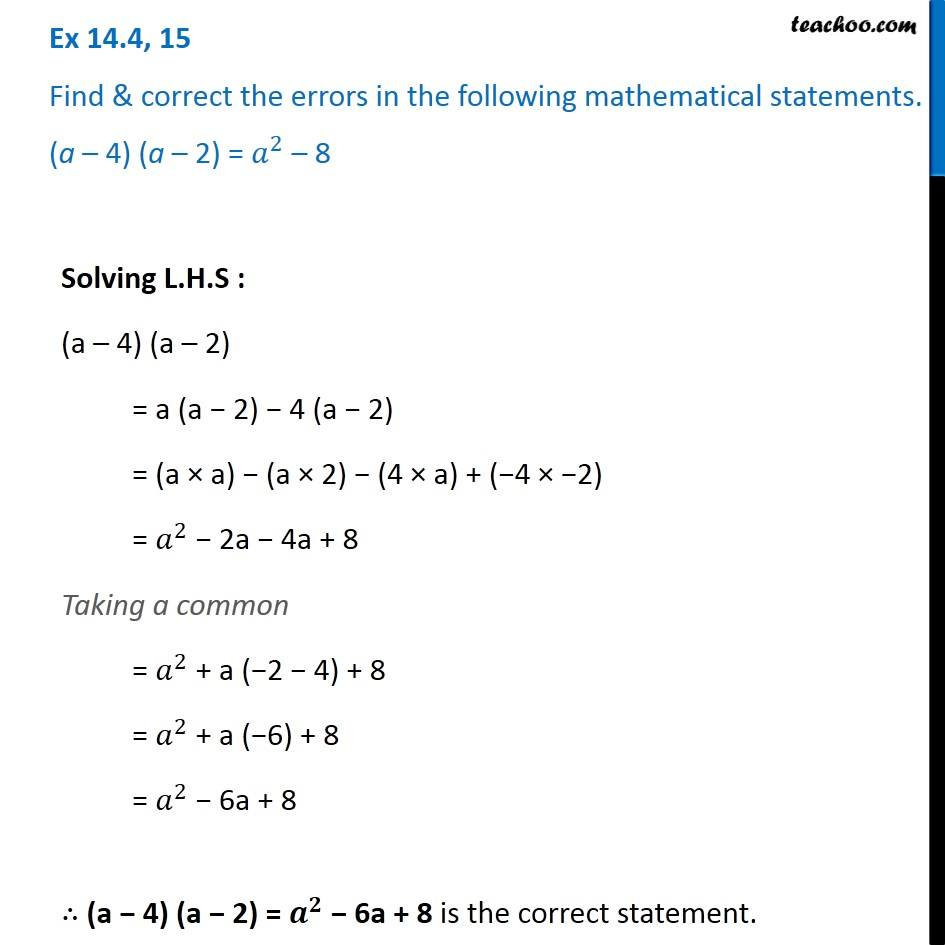Identify and correct errors in the sentences

Chapter 12 Class 8 Factorisation
Serial order wiseLearn in your speed, with individual attention - Teachoo Maths 1-on-1 Class

### Transcript

Question 15 Find & correct the errors in the following mathematical statements. (a – 4) (a – 2) = 𝑎^2 – 8 Solving L.H.S : (a – 4) (a – 2) = a (a − 2) − 4 (a − 2) = (a × a) − (a × 2) − (4 × a) + (−4 × −2) = 𝑎^2 − 2a − 4a + 8 Taking a common = 𝑎^2 + a (−2 − 4) + 8 = 𝑎^2 + a (−6) + 8 = 𝑎^2 − 6a + 8 ∴ (a − 4) (a − 2) = 𝒂^𝟐 − 6a + 8 is the correct statement.